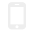APP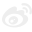微博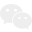微信A
B
C
D
E
F
G
H
J
K
L
M
N
P
Q
R
S
T
W
X
Y
Z

# 婚礼上那些令人惊艳的花，都有哪些寓意？

01
= 清漾林语 · 虞美人 =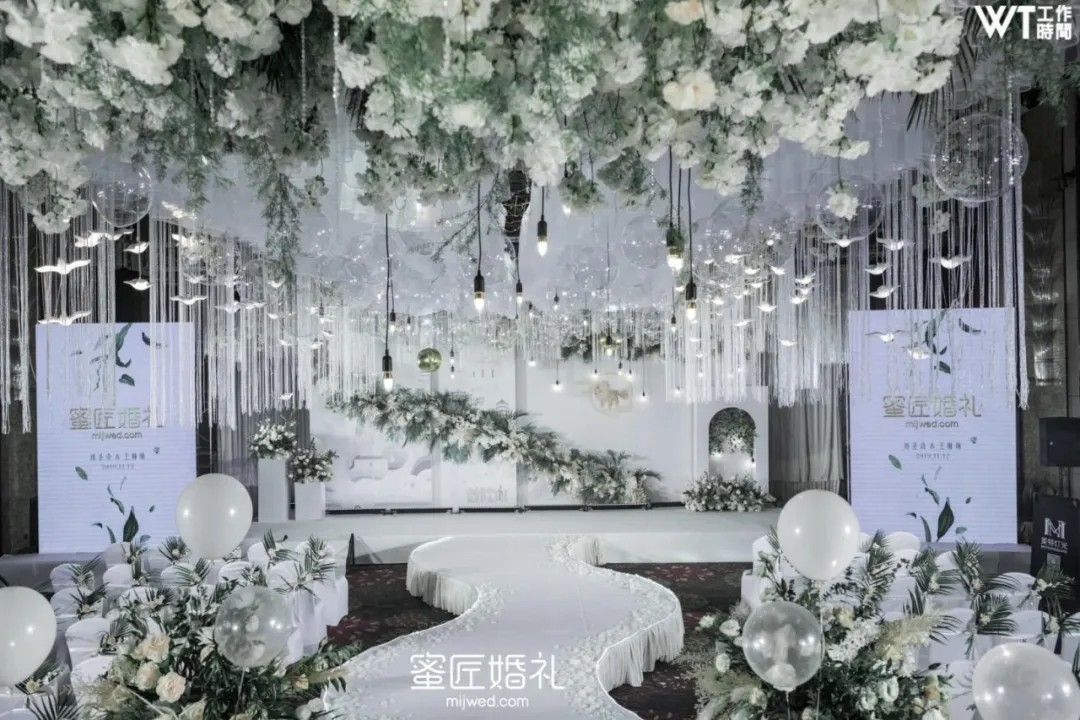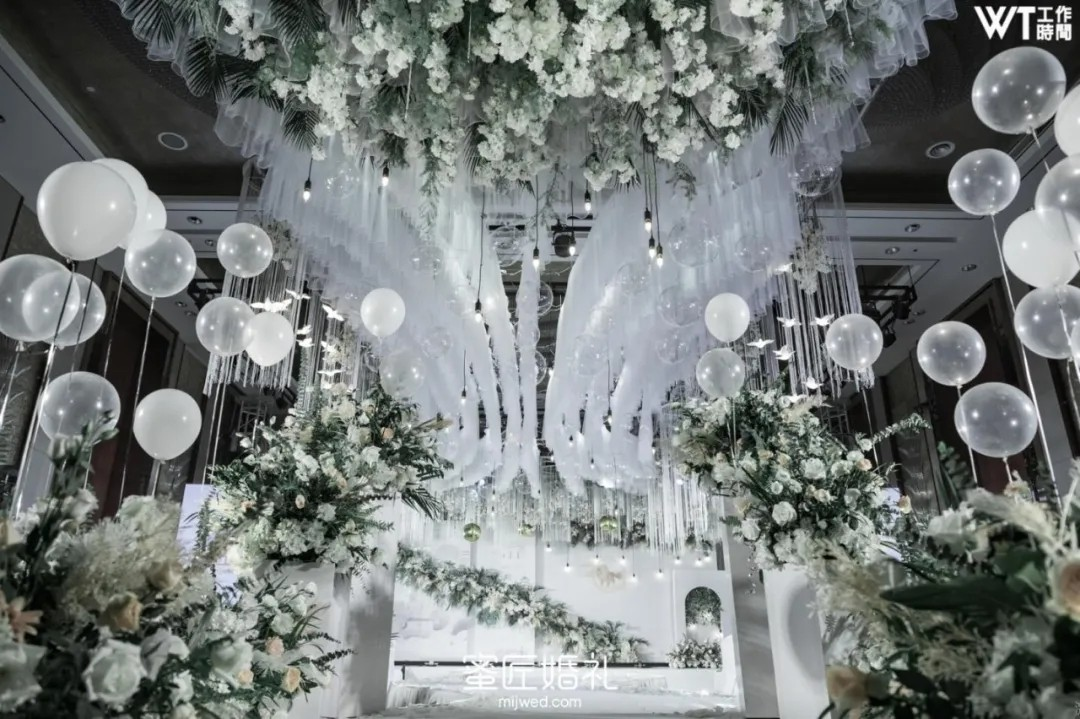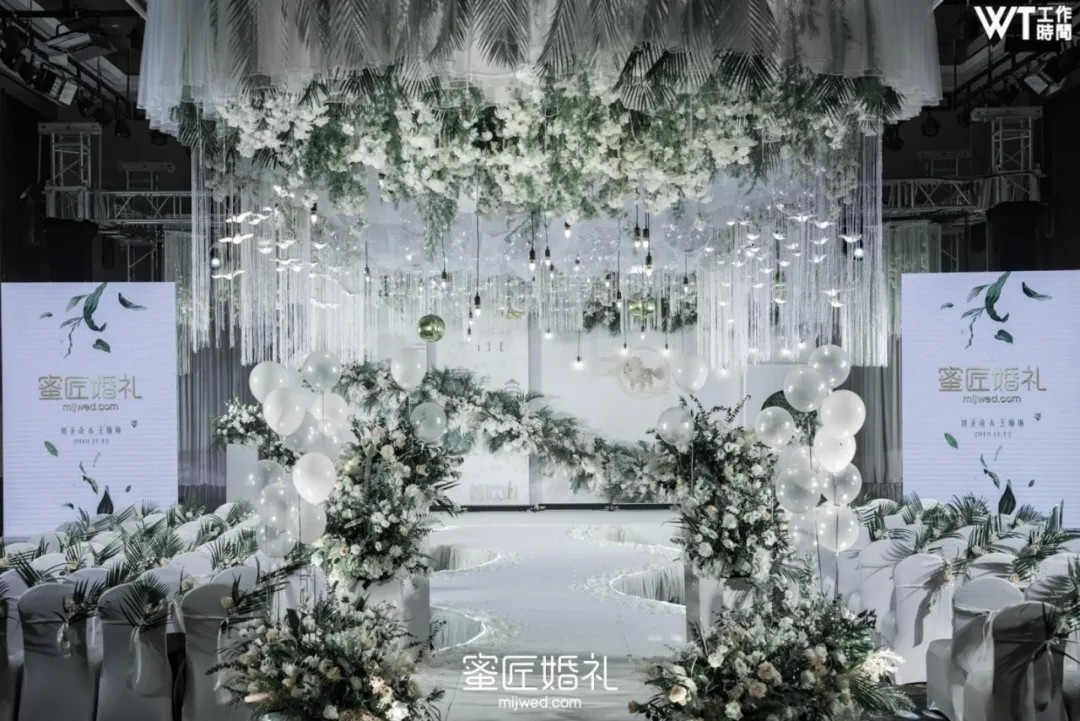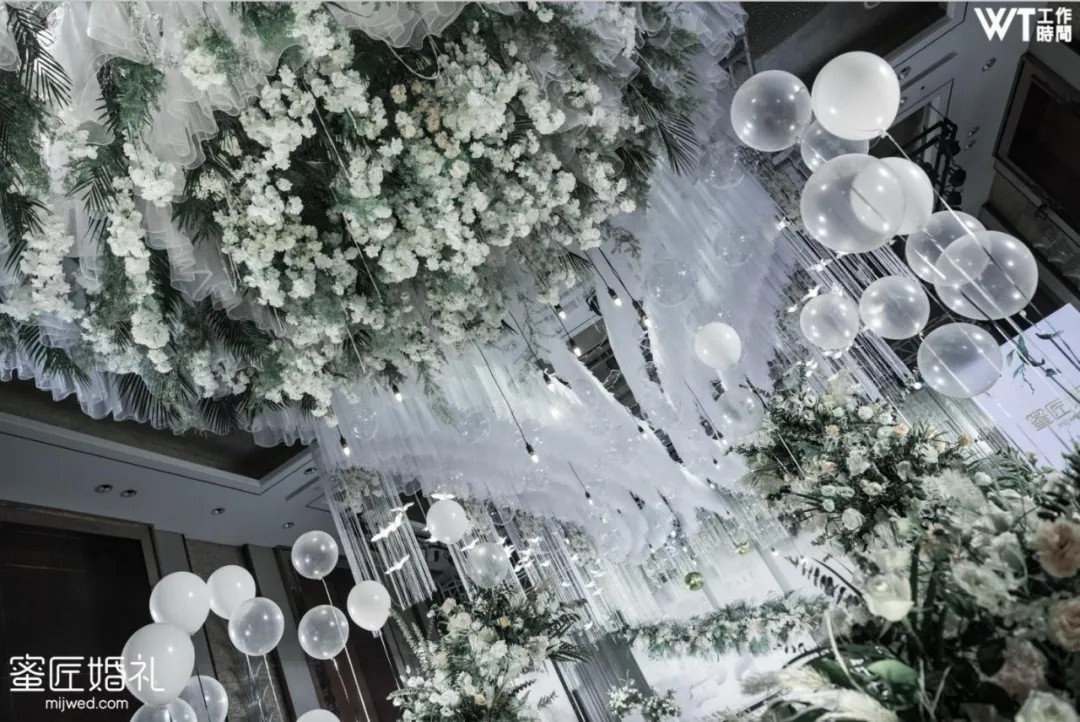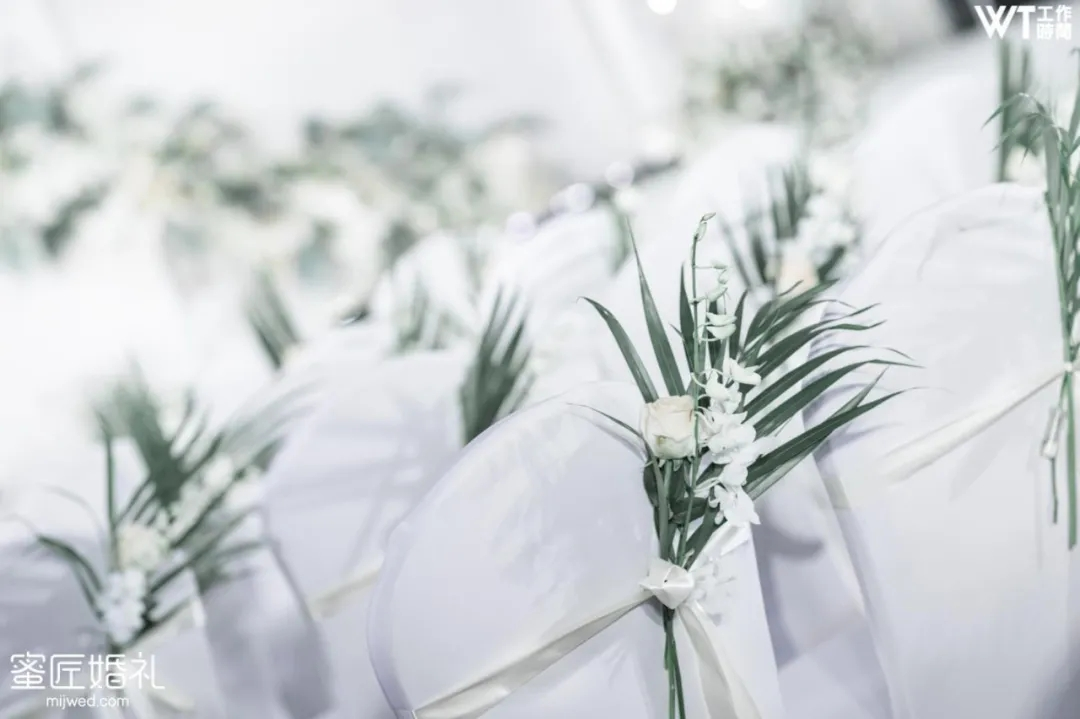02
= 如风 · 跳舞兰 =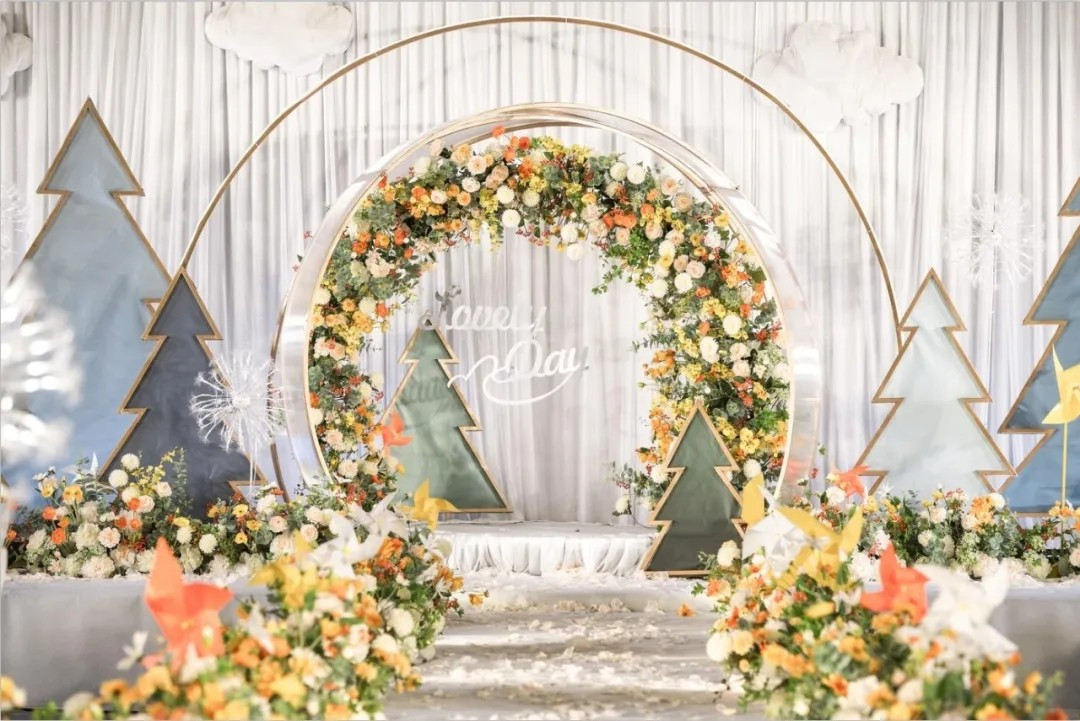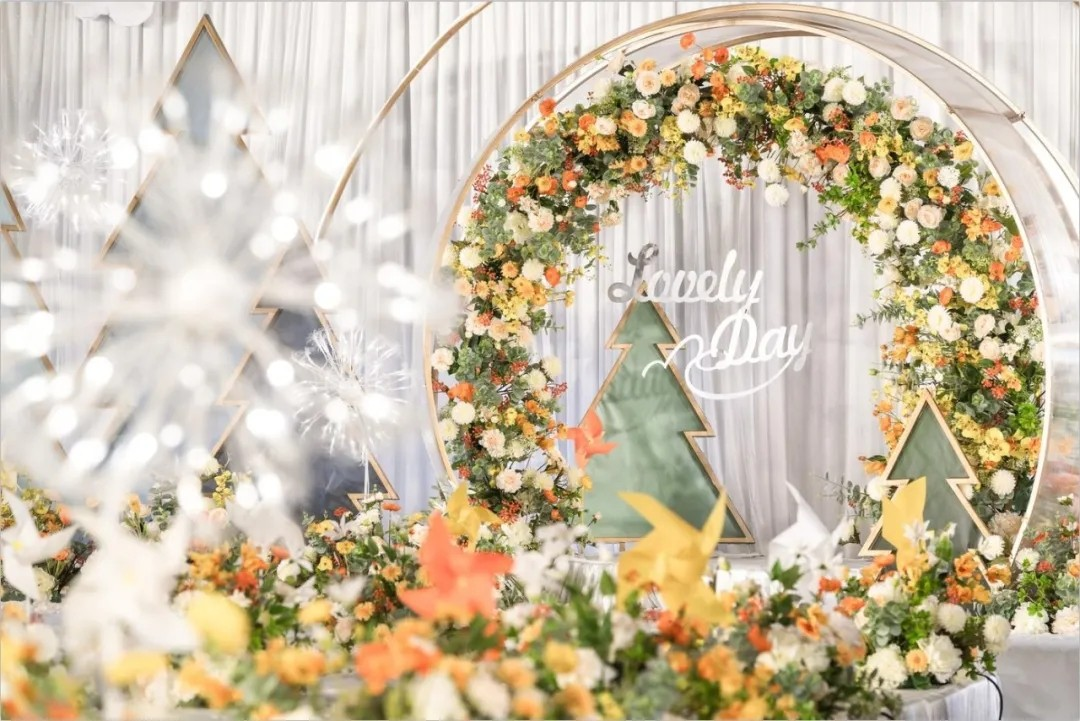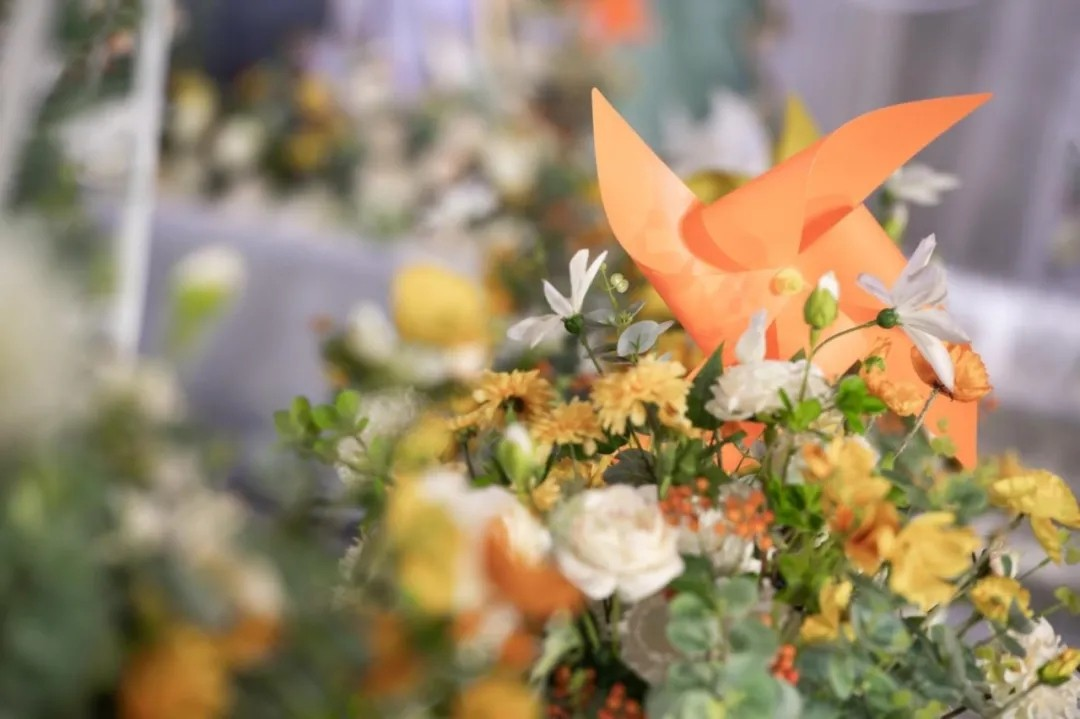03
= 伴侣 · 浅粉牡丹 =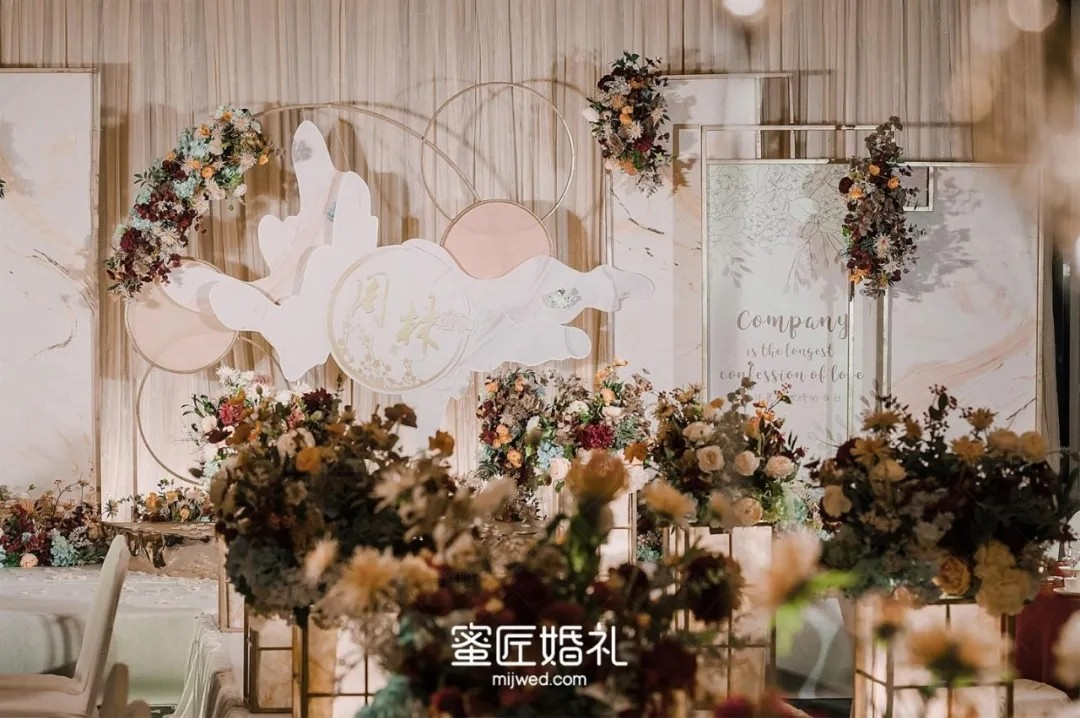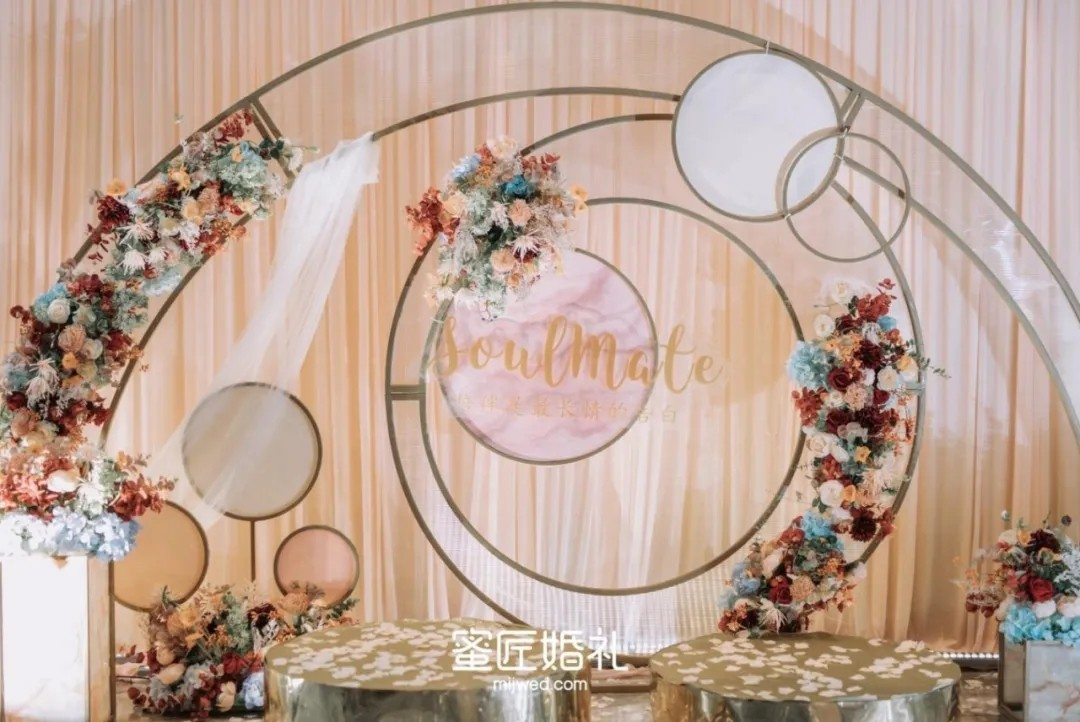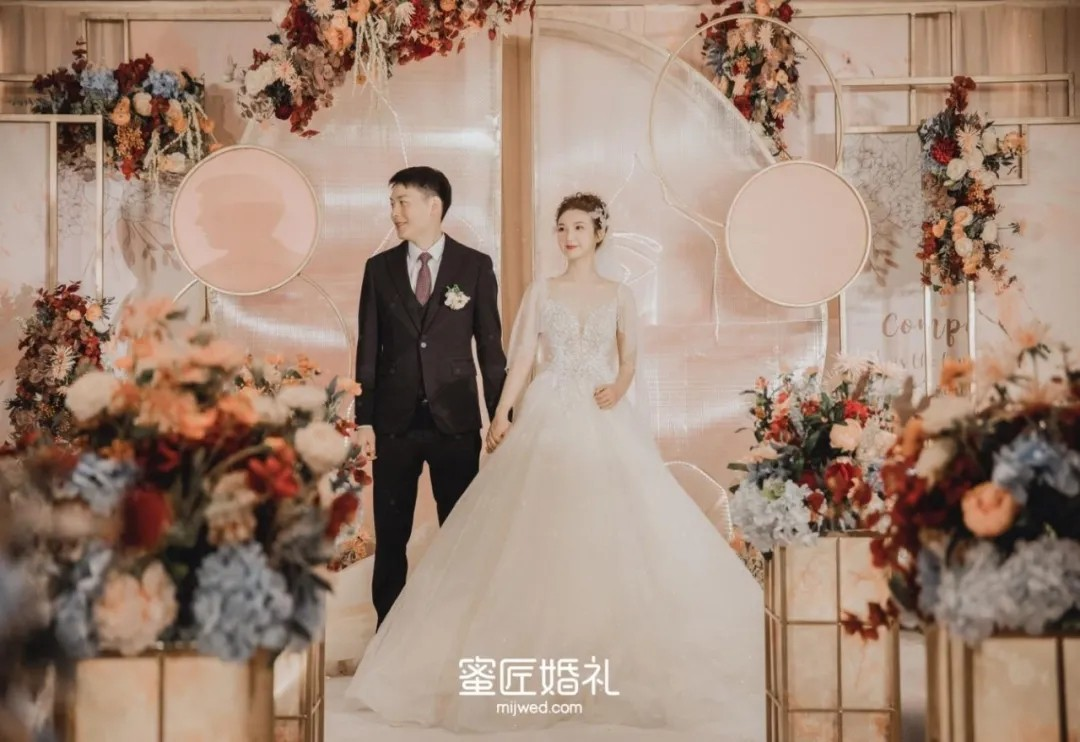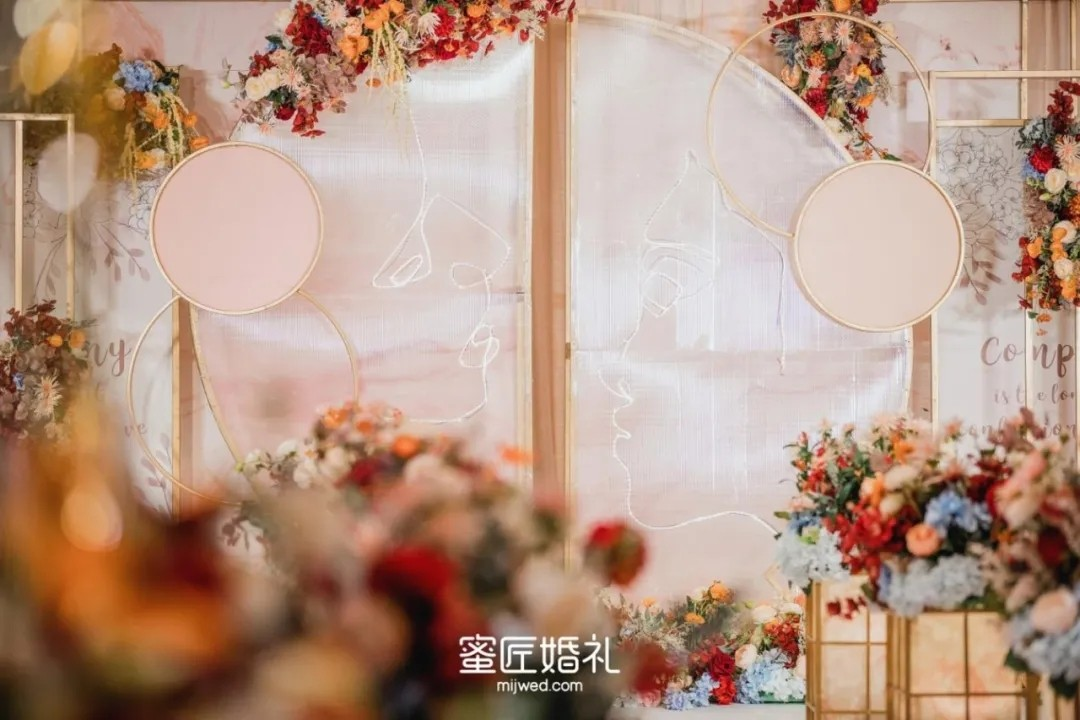04
= 小情歌 · 蟹爪兰 =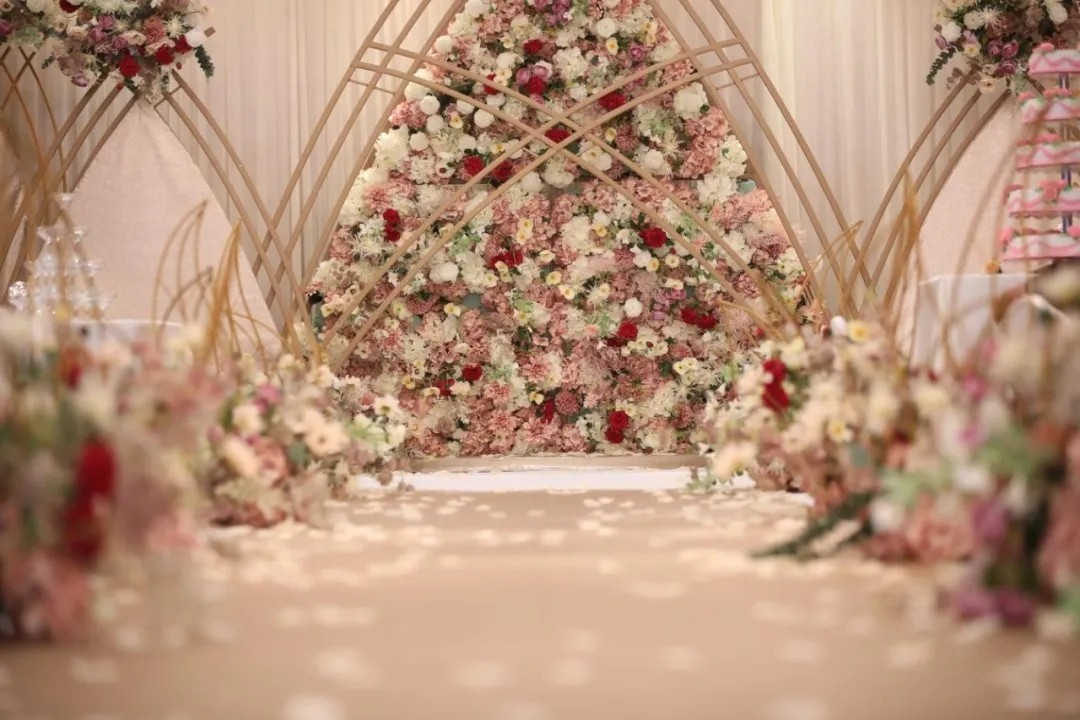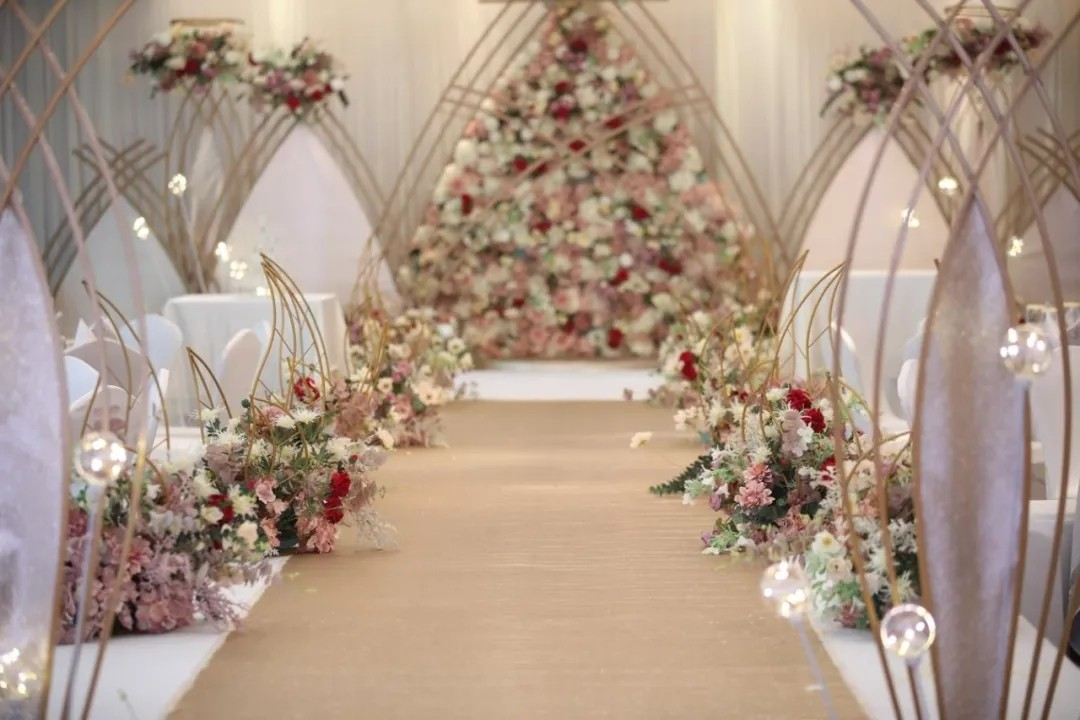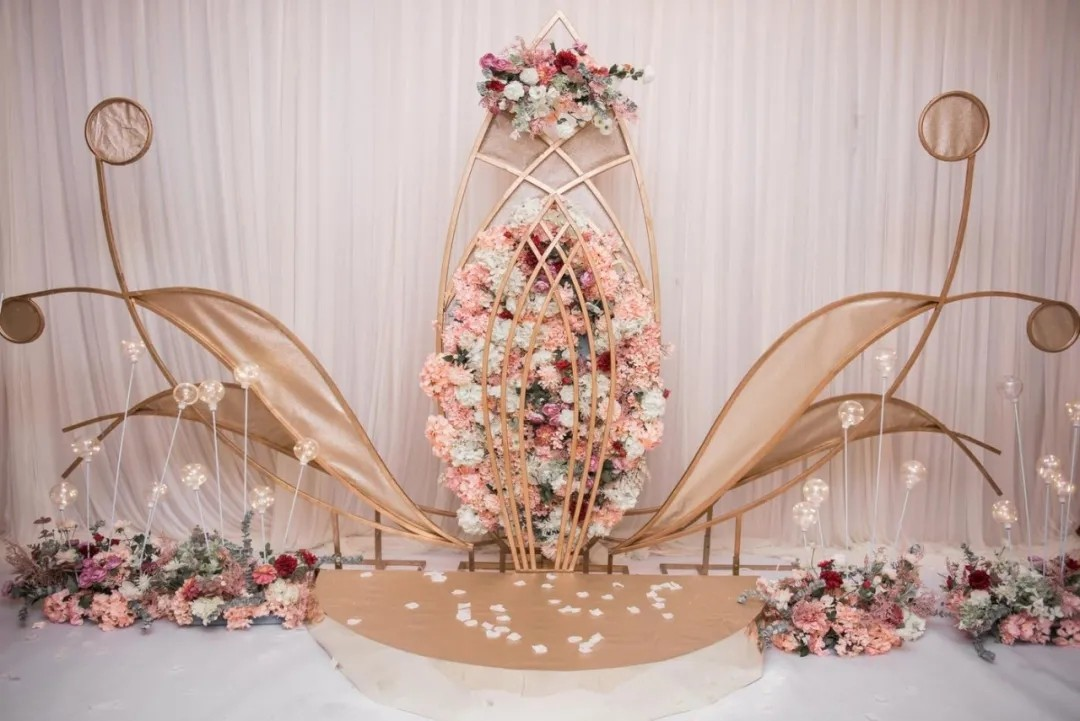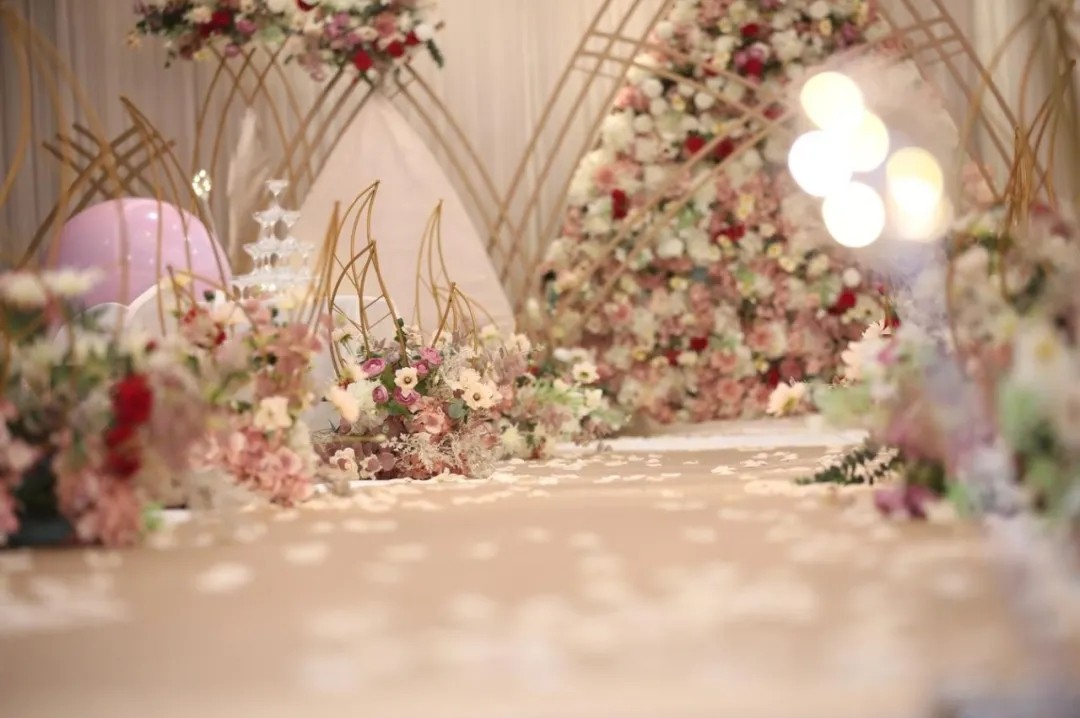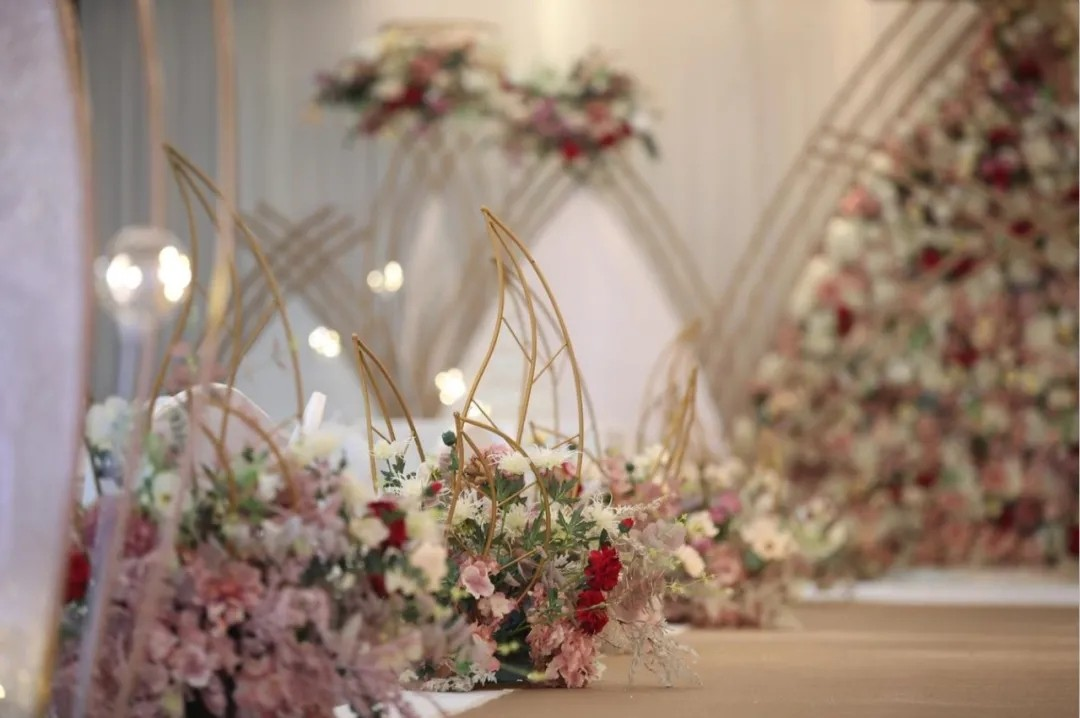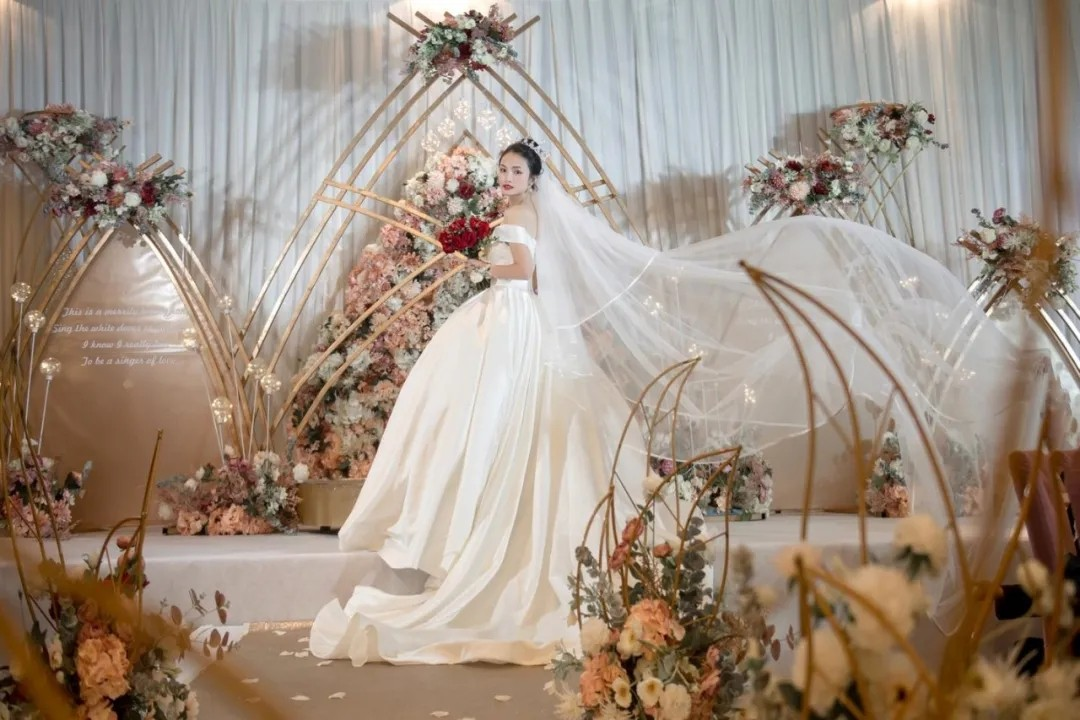05
= 情书 · 铃兰花 =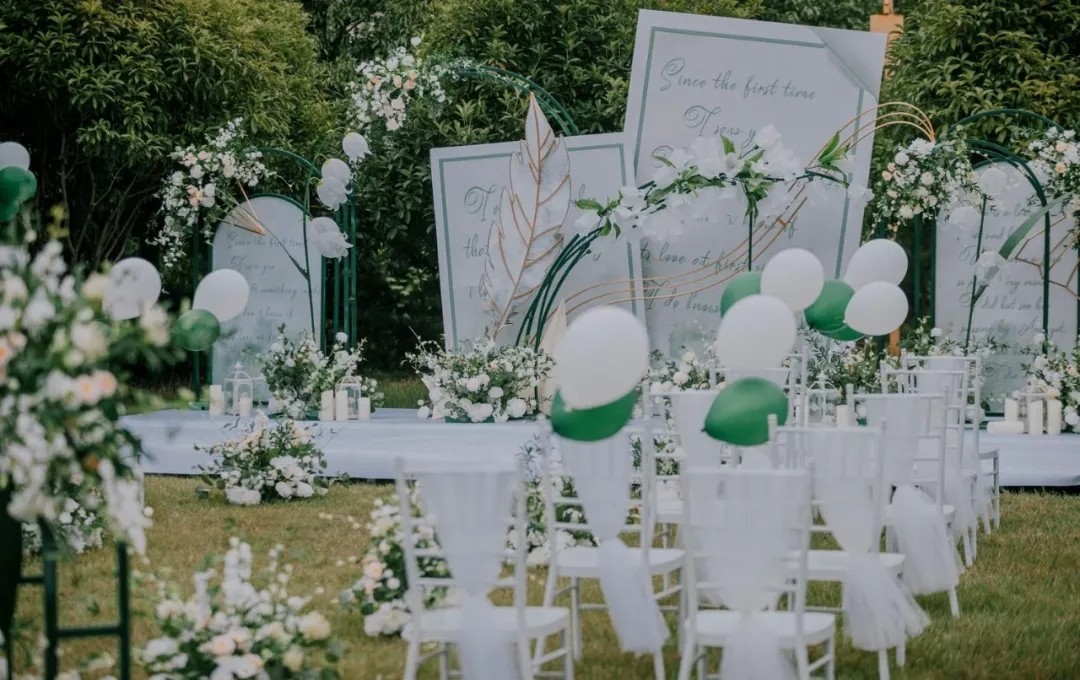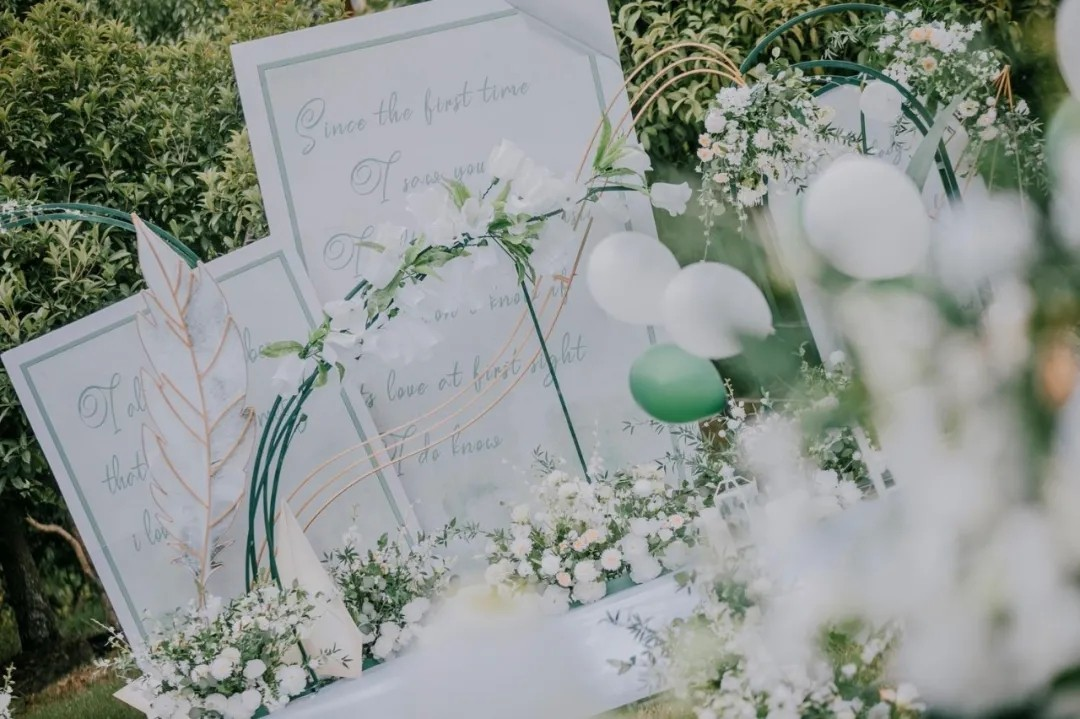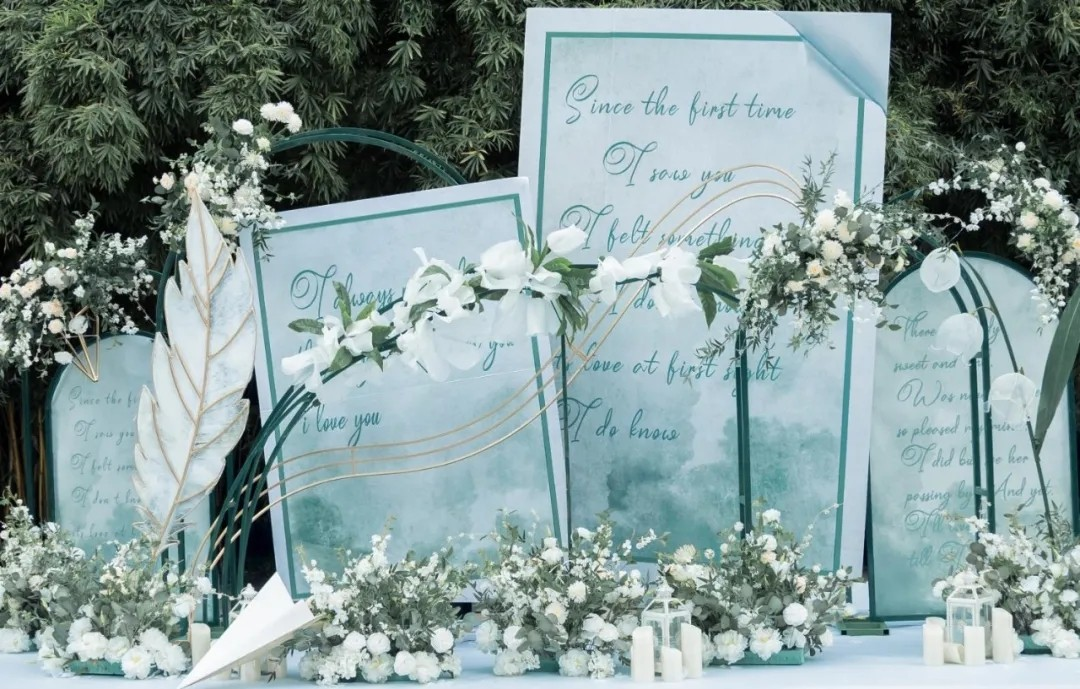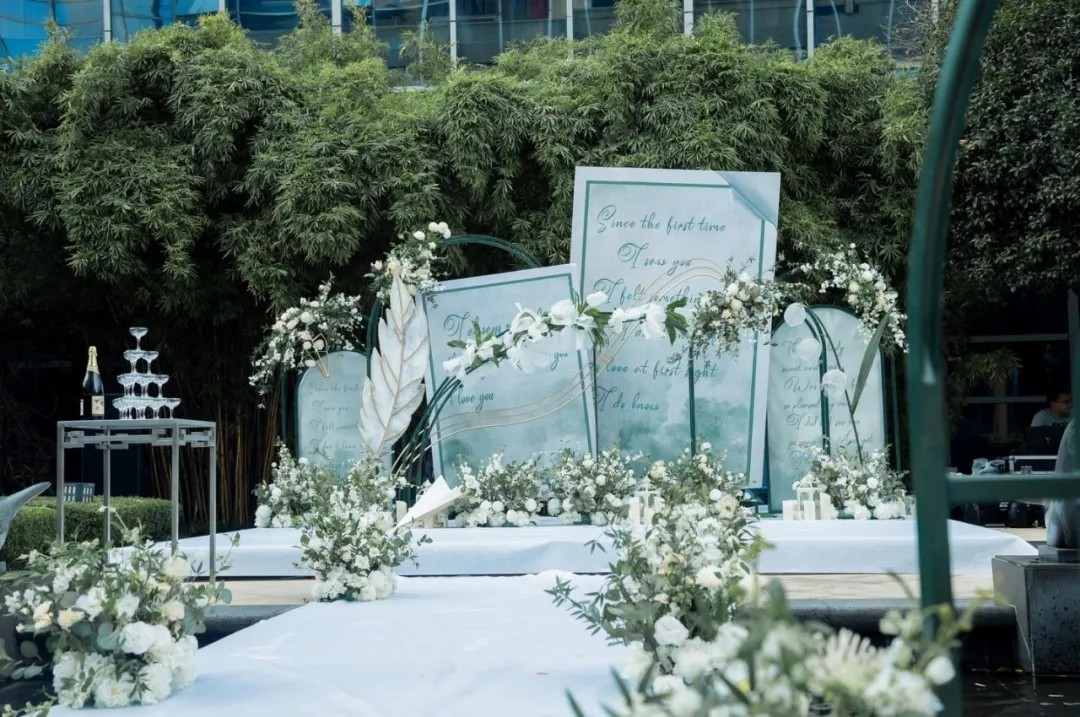06
= 克拉恋人 · 粉绣球 =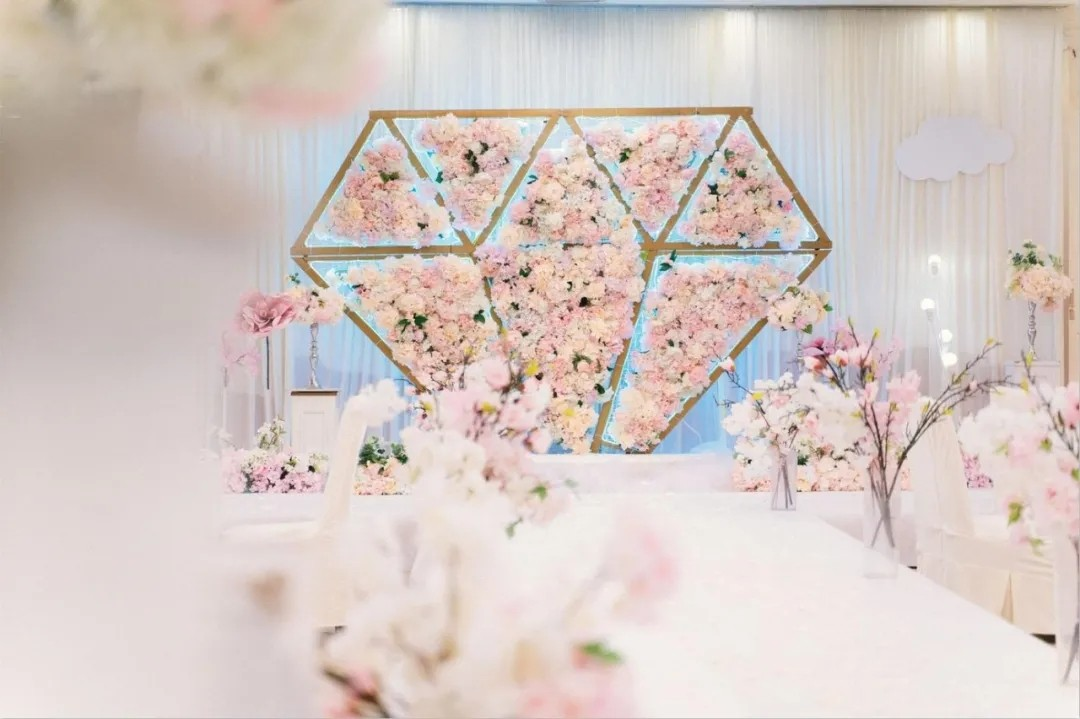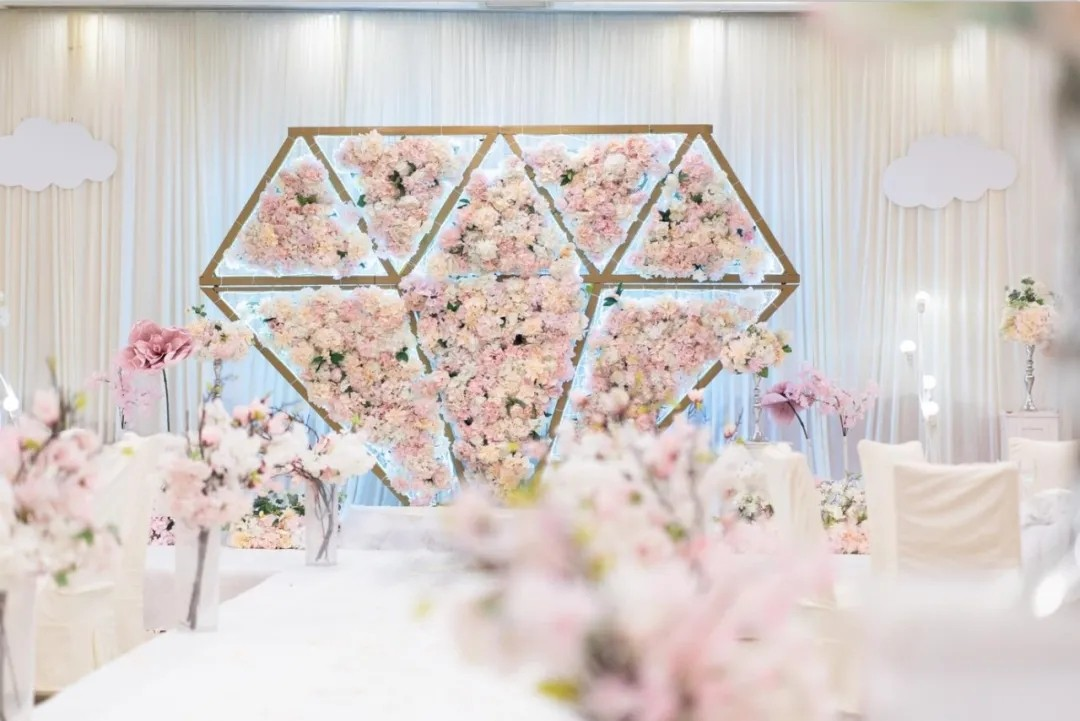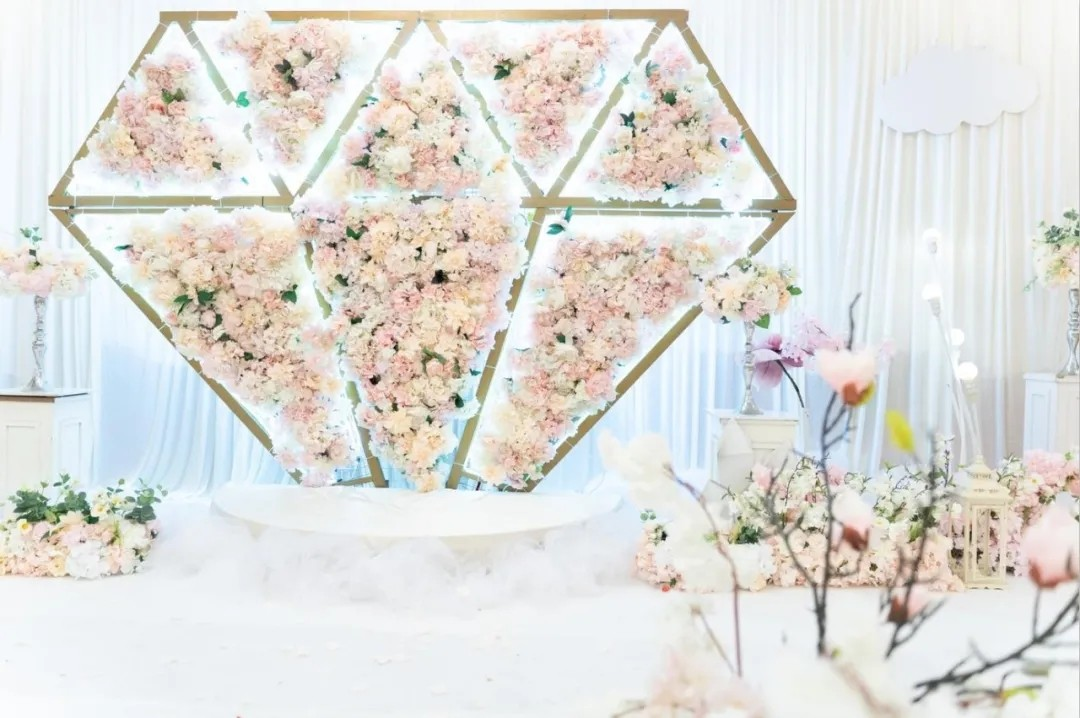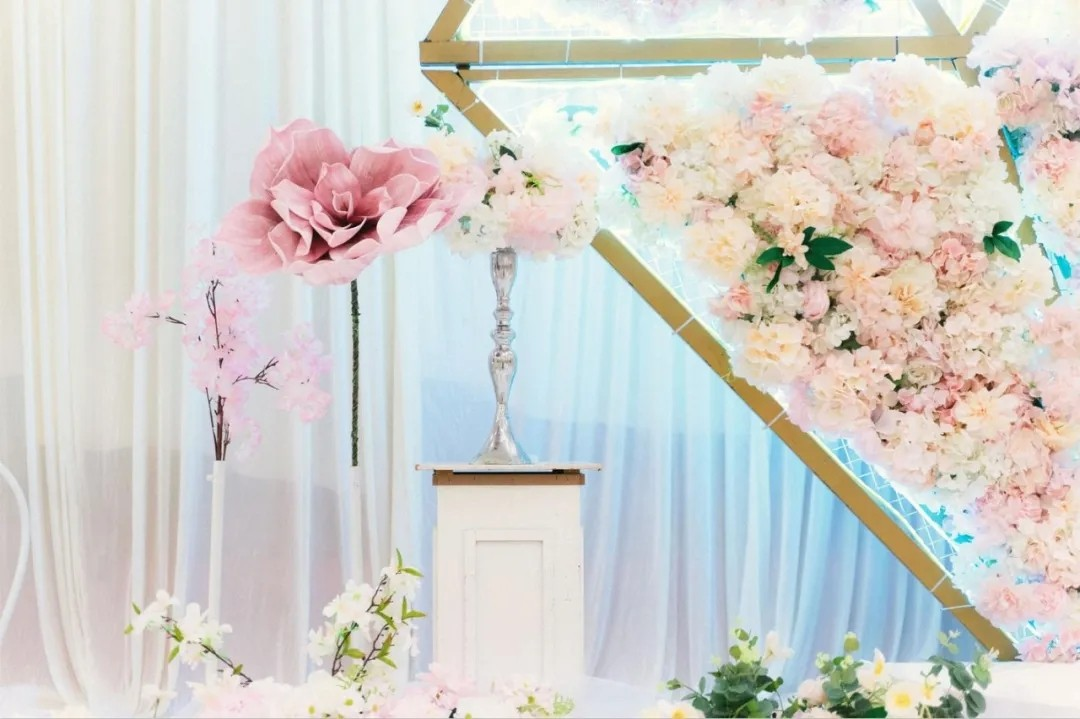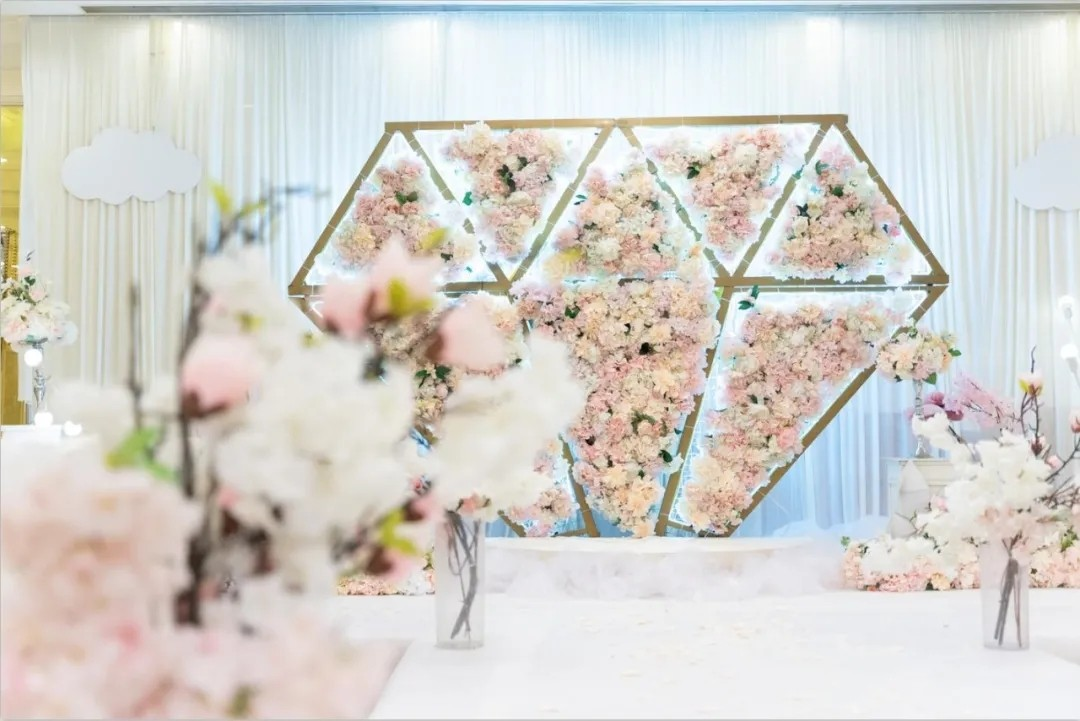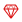热门主题婚礼 查看所有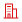热门婚宴酒店 查看所有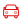热门婚车租赁 查看所有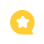最新婚礼布置文章编辑推荐